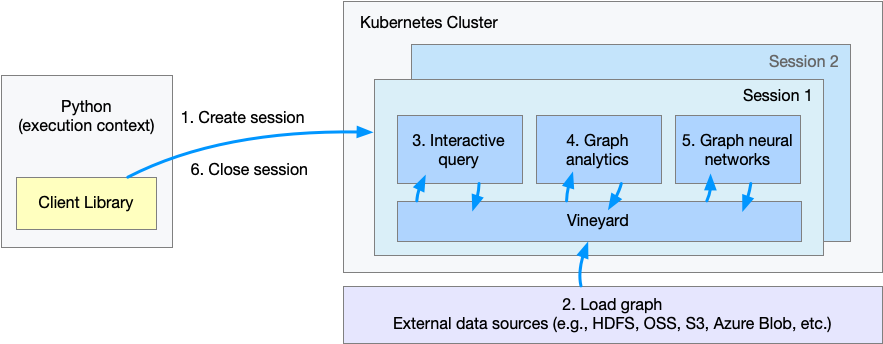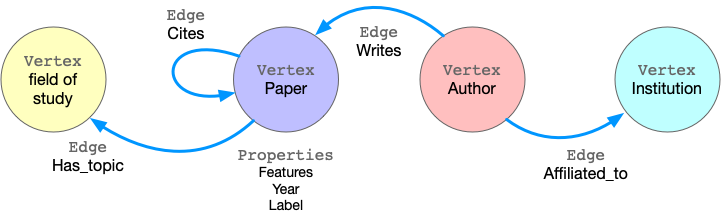# 快速上手

GraphScope 做为一站式图计算系统，可以处理图的交互式查询、图分析和图学习任务, 并且具有易于使用、高性能和良好的可扩展性等特点。

## 示例: 论文引用网络中的节点分类任务

ogbn-mag 数据集 是由微软学术关系图（Microsoft Academic Graph）的子集组成的异构图网络。 该图中包含4种类型的实体（即论文、作者、机构和研究领域），以及连接两个实体的四种类型的有向关系边。• 步骤 1. 建立一个会话（session），为 GraphScope 创建一个工作空间；

• 步骤 2. 定义和载入图；

• 步骤 3. 通过 gremlin 交互式查询图；

• 步骤 4. 执行图算法做图分析；

• 步骤 5. 执行基于图数据的机器学习任务；

• 步骤 6. 关闭会话。

## 创建会话

```import os
import graphscope

# assume we mount `~/test_data` to `/testingdata` in pods, and `ogbn_mag_small` within.
k8s_volumes = {
"data": {
"type": "hostPath",
"field": {
"path": os.path.expanduser("~/test_data/"),
"type": "Directory"
},
"mounts": {
"mountPath": "/testingdata"
}
}
}

sess = graphscope.session(k8s_volumes=k8s_volumes)
```

```sess = graphscope.session(k8s_volumes=k8s_volumes, k8s_service_type="LoadBalancer")
```

GraphScope 以属性图（property graph）建模图数据。属性图中，点和边都有一个标签（label），不同的标签有不同的属性（property）。 以 ogbn-mag 为例，下图展示了属性图的模型。```g = sess.g()
g = (
"author_affiliated_with_institution.csv",
label="affiliated",
src_label="author",
dst_label="institution",
)
"paper_has_topic_field_of_study.csv",
label="hasTopic",
src_label="paper",
dst_label="field_of_study",
)
"paper_cites_paper.csv",
label="cites",
src_label="paper",
dst_label="paper",
)
"author_writes_paper.csv",
label="writes",
src_label="author",
dst_label="paper",
)
)
```

```from graphscope.dataset import load_ogbn_mag

g = load_ogbn_mag(sess, "/testingdata/ogbn_mag_small/")
```

## 交互式查询

```# get the entrypoint for submitting Gremlin queries on graph g.
interactive = sess.gremlin(g)

# check the total node_num and edge_num
node_num = interactive.execute("g.V().count()").one()
edge_num = interactive.execute("g.E().count()").one()

# count the number of papers two authors (with id 2 and 4307) have co-authored.
papers = interactive.execute("g.V().has('author', 'id', 2).out('writes') \
.where(__.in('writes').has('id', 4307)).count()").one()
```

## 图分析

```# exact a subgraph of publication within a time range
sub_graph = interactive.subgraph("g.V().has('year', gte(2014).and(lte(2020))).outE('cites')")

# project the projected graph to simple graph.
simple_g = sub_graph.project(vertices={"paper": []}, edges={"cites": []})

ret1 = graphscope.k_core(simple_g, k=5)
ret2 = graphscope.triangles(simple_g)

# add the results as new columns to the citation graph
sub_graph = sub_graph.add_column(ret1, {"kcore": "r"})
sub_graph = sub_graph.add_column(ret2, {"tc": "r"})
```

## 图神经网络 (GNNs)

```# define the features for learning
paper_features = []
for i in range(128):
paper_features.append("feat_" + str(i))
paper_features.append("kcore")
paper_features.append("tc")

# launch a learning engine.
lg = sess.graphlearn(sub_graph, nodes=[("paper", paper_features)],
edges=[("paper", "cites", "paper")],
gen_labels=[
("train", "paper", 100, (0, 75)),
("val", "paper", 100, (75, 85)),
("test", "paper", 100, (85, 100))
])
```

```import graphscope.learning
from graphscope.learning.examples import GCN
from graphscope.learning.graphlearn.python.model.tf.trainer import LocalTFTrainer
from graphscope.learning.graphlearn.python.model.tf.optimizer import get_tf_optimizer

# supervised GCN.

def train(config, graph):
def model_fn():
return GCN(graph,
config["class_num"],
config["features_num"],
config["batch_size"],
val_batch_size=config["val_batch_size"],
test_batch_size=config["test_batch_size"],
categorical_attrs_desc=config["categorical_attrs_desc"],
hidden_dim=config["hidden_dim"],
in_drop_rate=config["in_drop_rate"],
neighs_num=config["neighs_num"],
hops_num=config["hops_num"],
node_type=config["node_type"],
edge_type=config["edge_type"],
full_graph_mode=config["full_graph_mode"])

graphscope.learning.reset_default_tf_graph()
trainer = LocalTFTrainer(model_fn,
epoch=config["epoch"],
optimizer=get_tf_optimizer(
config["learning_algo"],
config["learning_rate"],
config["weight_decay"]))
trainer.train_and_evaluate()

config = {"class_num": 349, # output dimension
"features_num": 130, # 128 dimension + kcore + triangle count
"batch_size": 500,
"val_batch_size": 100,
"test_batch_size":100,
"categorical_attrs_desc": "",
"hidden_dim": 256,
"in_drop_rate": 0.5,
"hops_num": 2,
"neighs_num": [5, 10],
"full_graph_mode": False,
"agg_type": "gcn",  # mean, sum
"learning_rate": 0.0005,
"weight_decay": 0.000005,
"epoch": 20,
"node_type": "paper",
"edge_type": "cites"}

train(config, lg)
```

## 关闭会话

```sess.close()
```